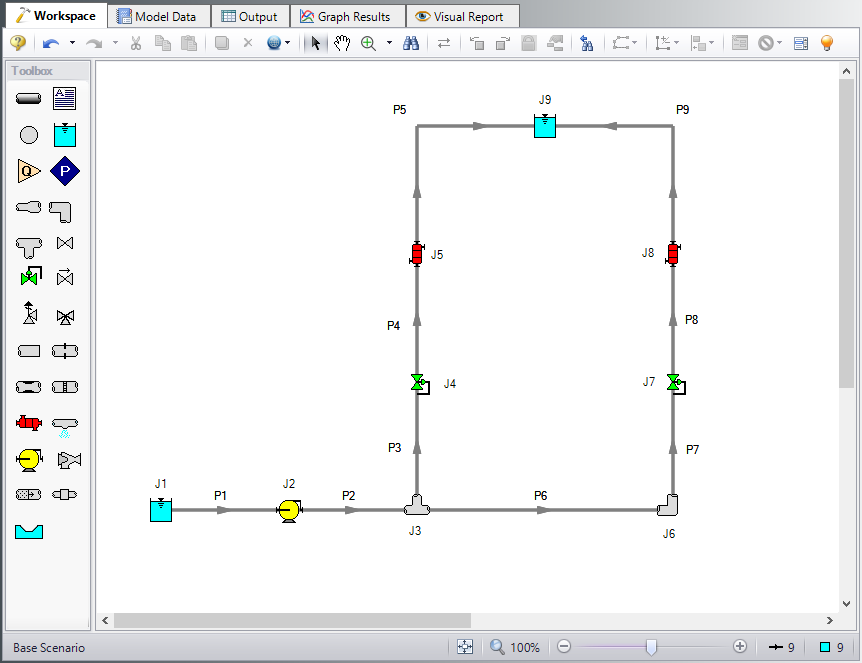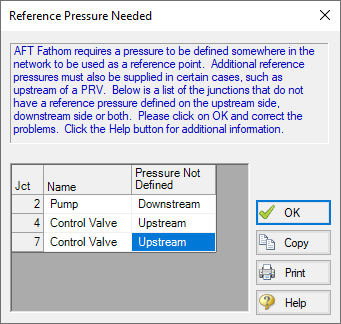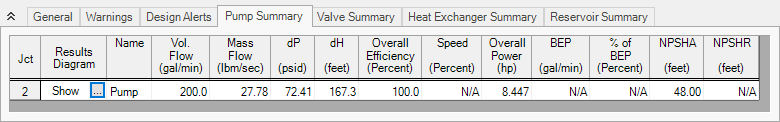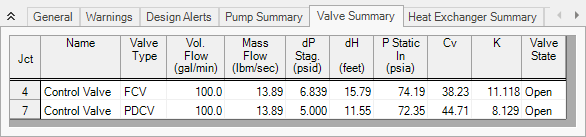## Pump Sizing and Selection with Flow Control Valves(English Units)

Summary

This example will demonstrate how to size and select pumps in a heat exchanger system with flow control valves. The system will pump water through a heat exchanger system from a supply tank at one elevation and pressure to a receiving tank at a higher elevation and pressure. There will be two flow control valves operating in parallel. The control valves require a minimum pressure drop of 5 psi in order to control the flow rate.

Topics Covered

• Using flow control valves

• Entering and changing pump data

Required Knowledge

This example assumes the user has already worked through the Beginner: Three Reservoir Problem example, or has a level of knowledge consistent with that topic. You can also watch the AFT Fathom Quick Start Video Tutorial Series on the AFT website, as it covers the majority of the topics discussed in the Three-Reservoir Problem example.

Model File

This example uses the following file, which is installed in the Examples folder as part of the AFT Fathom installation:

Step 1. Start AFT Fathom

From the Start Menu choose the AFT Fathom 12 folder and select AFT Fathom 12.

To ensure that your results are the same as those presented in this documentation, this example should be run using all default AFT Fathom settings, unless you are specifically instructed to do otherwise.

Step 2. Define the Fluid Properties Group

1. Open Analysis Setup from the toolbar or from the Analysis menu.

2. Open the Fluid panel then define the fluid:

1. Fluid Library = AFT Standard

2. Fluid = Water (liquid)

1. After selecting, click Add to Model

3. Temperature = 70 deg. F

Step 3. Define the Pipes and Junctions Group

At this point, the first two groups are completed in Analysis Setup. The next undefined group is the Pipes and Junctions group. To define this group, the model needs to be assembled with all pipes and junctions fully defined. Click OK to save and exit Analysis Setup then assemble the model on the workspace as shown in the figure below.Figure 1: Workspace for Pump Selection with Flow Control Valves Example

The system is in place but now the properties of the objects must be entered.

Pipe Properties

1. Pipe Model tab

1. Pipe Material = Steel - ANSI

2. Pipe Geometry = Cylindrical Pipe

3. Size = 2 inch

4. Type = STD (schedule 40)

5. Friction Model Data Set = Standard

6. Length =

Pipe Length (feet)
1 20
2 50
3 60
4 20
5 100
6 20
7 60
8 20
9 100

Junction Properties

1. J1 Reservoir

1. Name = Supply Tank

2. Liquid Surface Elevation = 5 feet

3. Liquid Surface Pressure = 10 psig

4. Pipe Depth = 5 feet

2. J2 Pump

1. Inlet Elevation = 0 feet

2. Pump Model = Centrifugal (Rotodynamic)

3. Analysis Type = Sizing

4. Parameter = Volumetric Flow Rate

5. Fixed Flow Rate = 200 gal/min

3. J3 Tee

1. Elevation = 0 feet

2. Loss Model = Simple

4. J4 & J7 Control Valve

1. Inlet Elevation = 0 feet

2. Valve Type = Flow Control (FCV)

3. Control Setpoint = Volumetric Flow Rate

4. Flow Setpoint = 100 gal/min

5. J5 & J8 Heat Exchanger

1. Inlet Elevation = 0 feet

2. Loss Model = Resistance Curve

3. Enter Curve Data =

Volumetric Pressure
gal/min psid
100 10

2. Curve Fit Order = 2

3. Click Generate Curve Fit Now

1. J6 Bend

1. Inlet Elevation = 0 feet

2. Type = Standard Elbow (knee, threaded)

3. Angle = 90 Degrees

2. J9 Reservoir

1. Name = Receiving Tank

2. Liquid Surface Elevation = 10 feet

3. Liquid Surface Pressure = 30 psig

4. Pipe Depth = 10 feet

ØTurn on Show Object Status from the View menu to verify if all data is entered. If so, the Pipes and Junctions group in Analysis Setup will have a check mark. If not, the uncompleted pipes or junctions will have their number shown in red. If this happens, go back to the uncompleted pipes or junctions and enter the missing data.

Step 4. Resolve Reference Pressure Needed

Click Run Model on the toolbar or from the Analysis menu.

The Reference Pressure Needed window will appear (see Figure 2). This window is displayed when a model is constructed that does not have a unique solution. A common occurrence of this is when a model contains one or more sections that are completely bounded by known flow rates.

In this case, because the pump has been designated as an assigned flow and the downstream control valves are flow control valves, there is no way to uniquely determine the pressure between the pump and the control valves without an additional reference pressure. The reference pressure is the pressure from which the other pressures in the system are derived. There can be more than one reference pressure, but there always has to be at least one.

For a more in-depth discussion of reference pressures, see the Fathom help file topic: Role of Pressure Junctions - Detailed DiscussionFigure 2: Reference Pressure Needed window

Open the J7 Control Valve Property window, and make the following changes.

1. Valve Type = Constant Pressure Drop (PDCV)

2. Control Setpoint = Pressure Loss

3. Pressure Drop = 5 psid

Step 5. Run the Model

Click Run Model on the toolbar or from the Analysis menu. This will open the Solution Progress window. This window allows you to watch as the AFT Fathom solver converges on the answer. This model runs very quickly. Now view the results by clicking the Output button at the bottom of the Solution Progress window.

Step 6. Examine the Output

We can look at the pump summary and see that the head at 200 gal/min needs to be at least 167.3 feet (see Figure 3). The control valves both have enough pressure drop to control flow (see Figure 4).Figure 3: Pump Summary windowFigure 4: Valve Summary window

Step 7. Enter a Pump Curve

Now we need to specify a real pump. We will enter in some pump data and create a pump curve with the curve fitting routines in Fathom. Use the Scenario Manager on the Quick Access Panel to create a child of the base scenario with a name of Pump Curve. Then in the Pump Curve scenario, open the Pump Properties window and enter the following data:

1. J2 Pump

1. Pump Model = Centrifugal (Rotodynamic)

2. Analysis Type = Pump Curve

3. Enter Curve Data =

gal/min feet
0 175
200 168
400 120
1. Curve Fit Order = 2

1. J7 Control Valve

1. Valve Type = Flow Control (FCV)

2. Control Setpoint = Volumetric Flow Rate

3. Flow Setpoint = 100 gal/min

Re-run the model and verify that the flow rate and pressure drop across the control valves is still within the limits.